﻿ 基于多分形谱及特征优选的说话人识别系统

# 基于多分形谱及特征优选的说话人识别系统Speaker Recognition System Based on Multifractal Spectrum Feature and Characters Selection Policy

Abstract: Speech is one kind of complicated non-linear signal, so traditional speech or speaker recognition system based on the linear theory is difficult to be further improved. In this paper, a new method based on the WTMM (wavelet transform modulus-maxima method) is proposed, which can facilitate the extraction of speech signals in the multifractal spectrum feature (MSF). The multifractal spectrum feature combined with the traditional linear features can obviously enhance performance of speaker recognition system. Experiment results show that 6-dimensional MSF combined with 13-dimensional MFCC and 16-dimensional LPC make error rate decrease to 1.2% in short speech speaker recognition. Then greedy algorithm is used to select 13 dimensional features from 101-dimensional features set. The experiment results show that the optimal feature selective method can eliminate disturbance of other redundant features, and obviously reduce the error rate, and improve the computational speed. The error rate decreases to 1.6%, and computation time decreases about 86%.

1. 引言

2. 多分形理论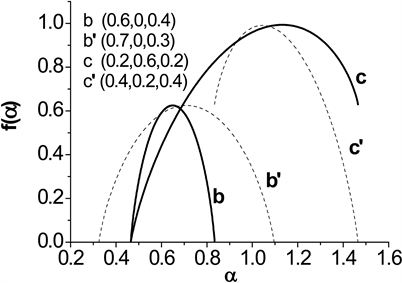Figure 1. Multifractal spectrum of different Cantor sets

1) 多分形谱中 $\alpha$ 表示的是不同的分形结构。在尺度变化过程中， $\alpha$ 越小表示结构的概率测度越大。

2) 多分形谱中的 $f\left(\alpha \right)$ 表示不同分形结构其数量与尺度的对数关系。 $f\left(\alpha \right)$ 越小说明对应分形结构数量较少。

3) 多分形谱的最大宽度 $\Delta \alpha ={\alpha }_{\mathrm{max}}-{\alpha }_{\mathrm{min}}$ 是多分形的一个重要参量，它描述了不同分形结构的差异程度，一般地讲， $\Delta \alpha$ 越大表明该信号包含的分形结构的差异度大。

4) ${f}_{\mathrm{max}}\left(\alpha \right)$ 代表了最主要的分形结构，可以表示信号的平均分形维数，反映了分形体的总体特性。其值越接近0，表示分形体形态越离散；其值越接近1，表示分形体形态越接近于线；其值越接近2，表示分形体形态越接近于面。

3. 语音多分形谱特征的计算

WTMM可以很方便的提取出一维信号的多分形谱结构。在固定尺度a时，对信号作小波变换，得到小波系数 ${W}_{f}\left(x,a\right)$，在x的邻域内，若有 $|{W}_{f}\left({x}_{0},a\right)|>|{W}_{f}\left(x,a\right)|$，则称 ${x}_{0}$ 为一个“局部极大值”点，极大值点的连线就是“极大模线族 $l\left(a\right)$ ”。定义配分函数：

$Z\left(q,a\right)=\underset{x\in l\left(a\right)}{\sum }\left({|{W}_{f}\left(x,a\right)|}^{q}\right)={a}^{\tau \left(q\right)}$ (1)

${W}_{f}\left(x,a\right)$ 在极大模线 $l\left(a\right)$ 上求和，得到q与 $\tau \left(q\right)$ 的关系， $\tau \left(q\right)$ 可以从 $Z\left(q,a\right)$ 和a的双对数曲线中求出。利用多分形热力学公式求取多分形谱：

$\left\{\begin{array}{l}D\left(h\right)=\underset{q}{\mathrm{min}}\left[qh-\tau \left(q\right)\right]\\ h=\frac{\partial \tau }{\partial q}\end{array}$ (2)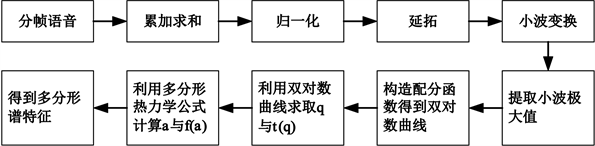Figure 2. Extraction process of the MSF

1) 累加求和。设某段分帧语音信号为： $x\left(i\right)$$i=1,2,\cdots ,N$，i为序号，N为帧长，利用公式 $s\left(n\right)=\underset{i=1}{\overset{n}{\sum }}x\left(i\right)$ 对信号累加求和，其中n为序号，与i一一对应。

2) 归一化。利用公式 $s\left(n\right)=s\left(n\right)/s\left(N\right)$，对 $s\left(n\right)$ 归一化，其中 $s\left(N\right)$ 是语音信号 $x\left(i\right)$ 累加和。归一化的目的的去除语音波形的幅度对计算的影响。

3) 延拓。延拓的目的是为了准确提取小波系数，特别是信号边缘部分的小波系数，公式如下：

$\left\{\begin{array}{l}S\left(n\right)=s\left(1\right),1 (3)

4) 小波变换。对 $S\left(n\right)$ 进行小波变换，本文采用墨西哥帽小波。

5) 提取小波极大值。遍历得到的小波系数，提取局部极大值。

6) 双对数曲线。根据公式(1)构造配分函数，其中求和只在局部极大值点处。由配分函数得到关于 $Z\left(q,a\right)$ 与a的双对数曲线，每一个q对应一条曲线。

7) 求取q与 $\tau \left(q\right)$ 。计算每条双对数曲线的斜率，用来估计 $\tau \left(q\right)$ 的值，从而得到q与 $\tau \left(q\right)$ 的关系。

8) 求取 $\alpha$$f\left(\alpha \right)$ 。利用多分形热力学公式(2)求取该语音段的MSF。

9) 简化MSF。由于计算出来的MSF维数较高，达到30维，因此根据本文第二部分对多分形谱图的分析，对MSF进行简化，只提取多分形谱中 ${f}_{\mathrm{max}}\left(\alpha \right)$${\alpha }_{\mathrm{min}}$${\alpha }_{\mathrm{max}}$ 三点信息来表征语音多分形信息，此三点信息可以反映语音的主要分形结构，分形结构的分布状态以及分布状态的不对称程度等信息。

4. MSF与传统特征结合实验

MSF与传统特征结合进行说话人识别实验，语音数据库选用的是TIMIT，数据库选用其中50人，每个人有10句语音，长度均为2秒。训练和识别分别选5句语音。所有的语音均为16,000 Hz采样，16位精度，提取特征参数时，MSF的帧长为32 ms，也就是512个采样点，帧移16 ms (256个采样点)；而MFCC (Mel Frequency Cepstrum Coefficient，梅尔频率倒谱系数)等传统特征的帧长和帧移均为16 ms (256个采样点)。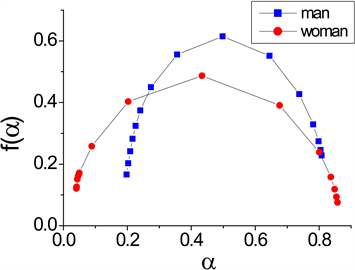Figure 3. The multifractal spectrum feature of Male and Female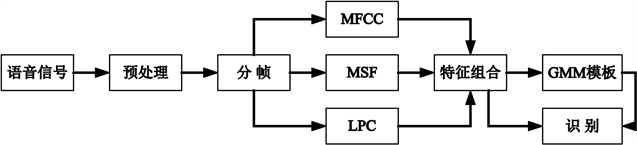Figure 4. Flow chart of speaker recognitionTable 1. False acceptance rate of different parameters combinations

5. 特征优选

5.1. 特征优选算法

1) 初始化，令 $i=0$，S为空，预定达到的误识率为R，预定挑选特征的数目为K；

2) 依次取 $j=1,2,\cdots ,W$，即从T中的取 ${t}_{j}$ 与S组合，作为训练和识别的特征 ${\tau }_{j}$，不同说话人的特征用 ${\tau }_{j,k}$ 表示；

3) 利用 ${\tau }_{j,k}$ 训练得到每个说话人的GMM参数 ${\lambda }_{j,k}$，并做识别实验，得到 ${\tau }_{j}$ 对应的误识率 ${r}_{j}$

4) $j$ 依次取1至W，得到一组误识率 ${r}_{j}$ 。令 $i=i+1$，取最小的误识率 $\mathrm{min}\left\{{r}_{j}\right\}$ 对应的特征 ${t}_{J}$ 为此轮挑选的特征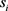，即 ${s}_{i}={t}_{J}$，将 ${s}_{i}$ 加入S中，即 $S=S+{s}_{i}$，并在T中去掉 ${\tau }_{J}$，即 $T=T-{t}_{J}$

5) 如果 $\mathrm{min}\left\{{r}_{j}\right\}>R$ 而且 $i，那么回到第(2)步，重复(2)到(4)步，如果 $\mathrm{min}\left\{{r}_{j}\right\}\le R$ 或者 $i\ge K$，则输出S。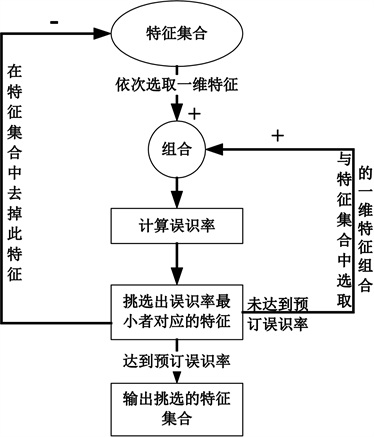Figure 5. Flow chart of feature selection method

5.2. 实验结果Table 2. False acceptance rate of the selection features from 101 dimensional features setTable 3. False acceptance rate of the selection features from 59 dimensional features setTable 4. False acceptance rate of the selection features from 30 dimensional MSFTable 5. False acceptance rate of the selection features from 29 dimensional MFCC+LPC

5.3. 计算时间分析

$O\left(L*{d}^{2}\right)+O\left({d}^{3}\right)+O\left(d*M\right)+O\left(I*L*d*M\right)+O\left(I*L*{M}^{2}\right)$

$O\left(N*L*{d}^{2}\right)+O\left(N*L*d*M\right)$

6. 结论

MSF的计算复杂度比较高，小波变换和利用配分函数求取MSF还没有比较成熟的快速算法，这大大影响了特征提取的计算时间，因此下一步研究重点是简化算法复杂度，开发快速算法。

 Seo, J.P., Kim, M.S., Baek, I.C., et al. (2004) Similar Speaker Recognition Using Nonlinear Analysis. Chaos, Solitons and Fractals, 21, 159-164.

 Petry, A. and Barone, D.A.C. (2002) Speaker Identification Using Nonlinear Dynamical Feature. Chaos, Solitons & Fractals, 13, 221-231.
https://doi.org/10.1016/S0960-0779(00)00260-5

 Fan, Y.L., Yi, L. and Tong, Q.Y. (2008) Speaker Gender Identification Based on Combining Linear and Nonlinear Features. 7th World Congress on Intelligent Control and Automation, Chongqing, 25-27 June 2008, 6739-6744.

 Hou, L.M. and Wang, S.Z. (2004) Generalized Dimensions Applied to Speaker Identi-fication. Biometric Technology for Human Identification, Orlando, FL, 12-13 April 2004, 555-560.
https://doi.org/10.1117/12.542828

 Arneodo, A., Audit, B., Bacry, E., et al. (1997) Thermodynamics of Fractal Signals Based on Wavelet Analysis: Application to Fully Developed Turbulence Data and DNA Sequences. Physica A: Statistical Mechanics and its Applications, 254, 24-45.
https://doi.org/10.1016/S0378-4371(98)00002-8

 Kestener, P. and Arneodo, A. (2008) A Mul-tifractal Formalism for Vector-Valued Random Fields Based on Wavelet Analysis: Application to Turbulent Velocity and Vorticity 3D Numerical Data. Stochastic Environmental Research and Risk Assessment, 22, 421-435.
https://doi.org/10.1007/s00477-007-0121-6

 李彤, 商朋见. 多重分形在掌纹识别中的研究[J]. 物理学报, 2007(8): 4393-4400.

 叶吉祥, 王聪慧. 多重分形在语音情感识别中的研究[J]. 计算机工程与应用, 2012, 48(13): 186-189.

 刘婷婷. 基于因子分析的与文本无关的说话人辨认方法研究[D]: [硕士学位论文]. 合肥: 中国科学技术大学, 2014.

 张庆芳, 赵鹤鸣, 龚呈卉. 基于因子分析和特征映射的耳语说话人识别[J]. 数据采集与处理, 2016, 31(2): 362-369.

 张翔. 基于因子分析的鲁棒性说话人识别技术研究[D]: [博士学位论文]. 北京: 中国科学院研究生院, 2011.

 徐利敏, 唐振民, 何可可, 等. 基于加权特征补偿变换的说话人识别仿真研究[J]. 系统仿真学报, 2008, 20(3): 616-619.

 俞一彪, 芮贤义, 许允喜. 说话人语音特征子空间分离及识别应用[J]. 电路与系统学报, 2008(1): 7-11.

 Kim, M.-S., Yu, H.-J., Kwak, K.-C., et al. (2006) Robust Text-Independent Speaker Identification Using Hybrid PCA & LDA. Mexican International Conference on Artificial Intelli-gence, Mexico, 13-17 November 2006, 1067-1074.

 毛勇, 周晓波, 夏铮, 等. 特征选择算法研究综述[J]. 模式识别与人工智能, 2007(2): 211-218.

Top